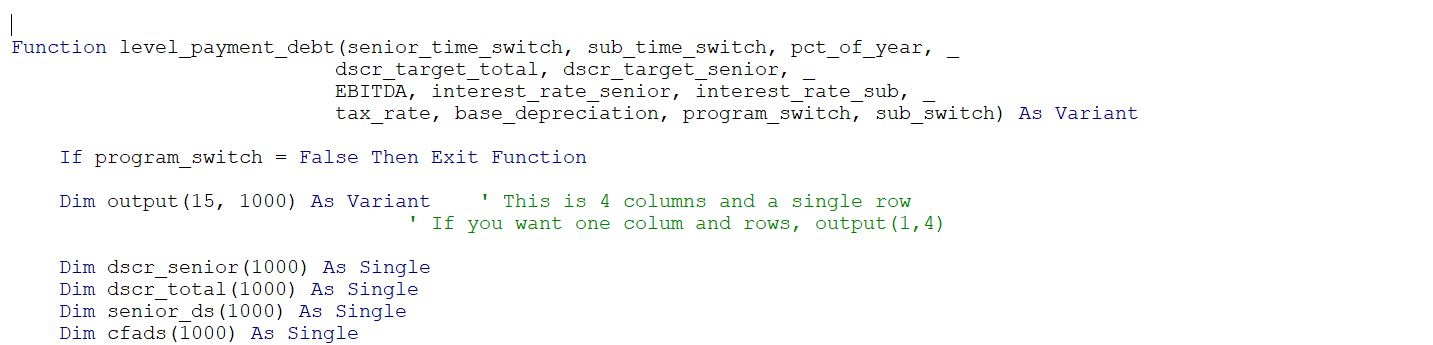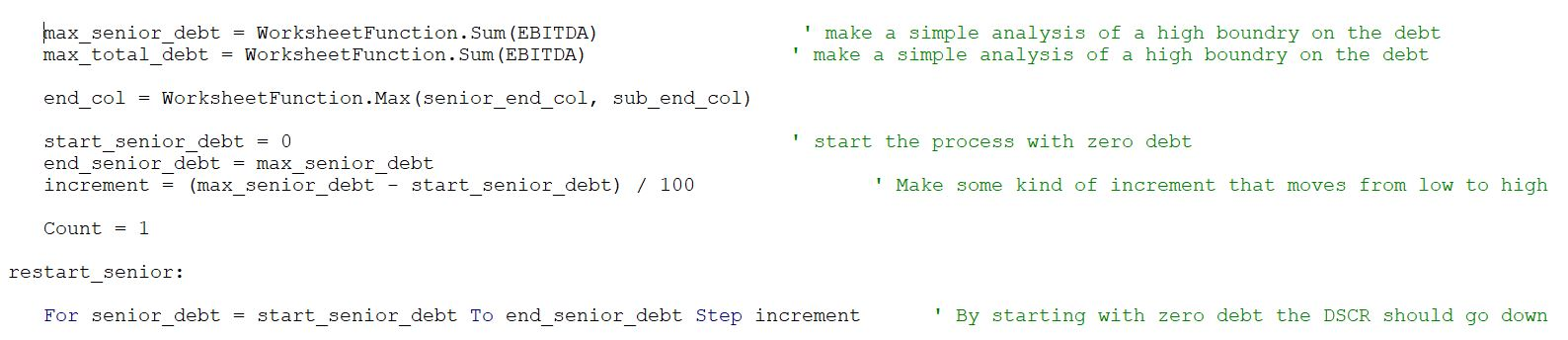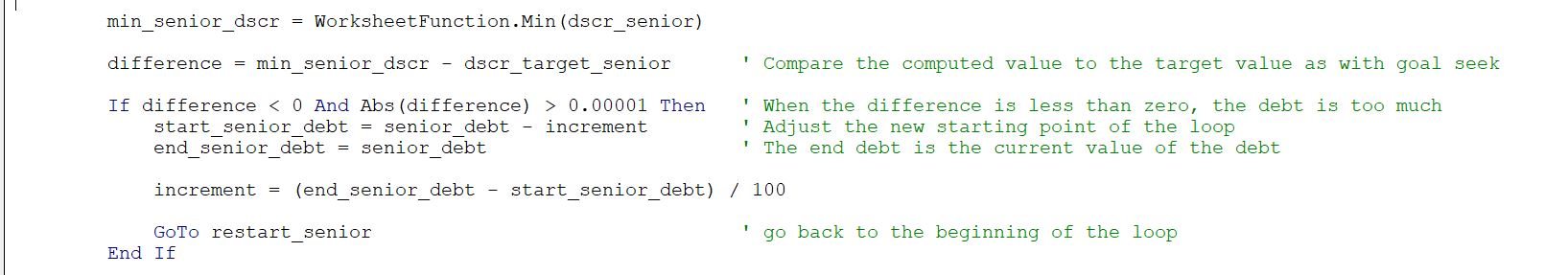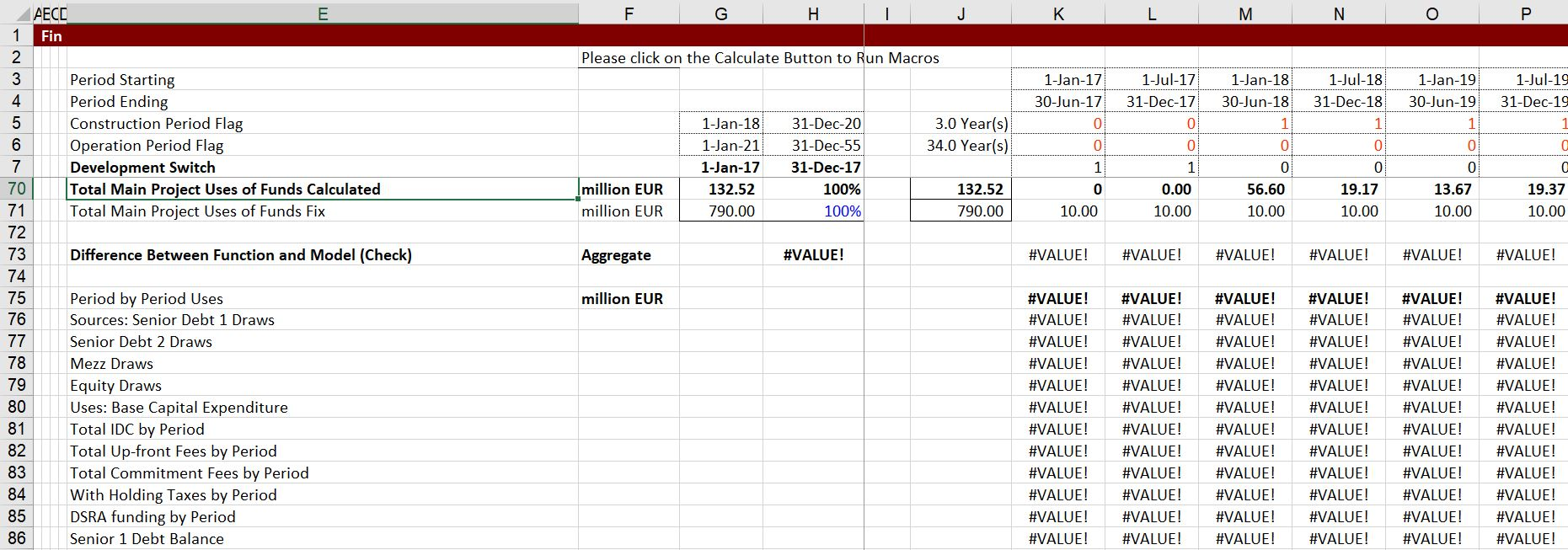# Dynamic Goal Seek

The goal seek is a wonderful tool in excel. But sometimes you may want to have the goal seek work on a dynamic basis, meaning that you do not want to go to the data menu and then hit the what if analysis and enter the target cell etc. A dynamic goal seek function is where you make a function that automatically changes to an output variable that comes from a goal seek when you change one of the inputs. The process of making a dynamic goal seek is similar to resolving a circular reference with a user-defined function. The similarity is that you need to re-program equations in excel and that you must do this with a user-defined function that has so kind of iteration loop. A dynamic goal seek can be useful in project finance models when you have a an equal installment or a level repayment. If you later want to operate another goal seek that derives prices or capital expenditures this can be very useful. For corporate finance, the dynamic goal seek could be useful in deriving the equity capital injection to reach a target capital structure so that you do not have to run the solver. In addition, the when you are backing out the cost of capital and the terminal value is a function of the cost of capital itself this technique can be very useful.

## Introduction to Dynamic Goal Seek

As with any goal seek function, you input a target amount for a particular cell that has a formula (e.g. the DSCR in project finance or the P/E ratio in cost of capital analysis) and you define the cell that changes to reach the target (in excel this must be an input). The basic idea of a dynamic goal seek is to make a FOR and NEXT loop where the loop changes the changing cell value. When making this loop, you work around the loop with a step increment. When the target value is reached, you stop the loop. This all sounds simple, but it can be a pain as I try to explain below. The objective of this exercise and the subsequent subjects is to try and make the process easier. So here are the steps:

Step 1: Standard UDF Input and Output Issues

As with other UDFs, you cannot use excel RANGE values. Instead you must input the target and all of the values (such as interest rates and EBITDA in the DSCR exmaple) that will be necessary to compute the value to be tested against the target. You can also define you dynamic goal seek as an array variable so you can evaluate why your dynamic goal seek is not working. An example of reading in variables and defining the UDF as an array is illustrated below. The key variable for the goal seek are the DSCR targets.Step 2: Define the start and end changing values for the loop.

The process involves putting a starting point and ending point for the first go around of the loop. You must make a starting point that can be difficult. In the example below, the starting debt is zero (assuming you will not have negative debt) and the ending debt is the sum of all of the EBITDA without any interest rate which is a very high value. Coming up with starting and ending points can be difficult and sometimes you may have to be creative. I wish I had a rule that always works, but you can probably start with zero and then come up with some way to define the maximum. It is also a pain to define the starting increment. I do something quite arbitrary.Step 3: Make calculations for evaluating the target value.

As with UDF’s that are made for resolving circular references and getting out of a circular loop, you must put all of the calculations inside the loop with the STEP Increment. Often, you will have a second loop that works from the beginning of the debt to the end of the debt.In the example below, the debt balance is computed and then used to compute repayments and debt service and the the DSCR (which is maintained in an array).Step 4: In the loop evaluate whether the target is met (or exceeded).

In the loop there must be some test as to whether the target is met or exceeded. For example if the debt starts with 0, the DSCR will be very very high (infinitiy). As you increase the amount of the debt, the DSCR will go down. Eventually, the DSCR will be below the target DSCR. When the target is met, you should go back and re-try the loop with smaller increments. When you re-do the loop, you should go back and increase the amount of the debt to the prior increment and define the end with the current value that made the DSCR too low. For example if a value of debt of 200 makes the DSCR too low and the increment is 10, then you should go back and make the debt 190 and the high value 200. The increment can be the value of 10 divided by 100. Then the next loop will be much more precise. If you look above, there is a label named RESTART_SENIOR:. You can go back there and then re-try the loop. This illustrated in the excerpt below where a difference between the computed DSCR and the target DSCR is made.Step 5: Test whether you have succeeded in meeting the target and exit the loop.

After you put the test to evaluate whether the target is exceeded, you can see if the you are finished, because the changing value results in a number very close to the target. Not that I think you should be careful and not put a test that is exactly equal to zero. This is like the option in excel where you enter the convergence and the iterations. You should do something similar with a dynamic loop. In this case you should make a counter variable because of the danger of large loops. You should also make a test that allows you to exit the loop and report the outputs. When you do this, you are deciding whether to continue and try again as in step 2 or alternatively to end the loop. The test should be included in both sections. Note that the above excerpt has a value of .00001 and if the absolute value of the difference is more than this value, you should try again. But when the difference is less than this very small value, you should end the loop. This is demonstrated in the the excerpt below.## Fundamental Ideas of Dynamic Goal Seek

In the example that is documented with the video below, I made a really simple example where you add two numbers and then make a goal seek to see what the first number has to be to derive a target sum. For example, you input a value of 2 and you want to see what value, when added to 2, will give you a target. If you were doing this with a goal seek, you would make a calculation with 2 + 2 = 4. Then you could put in a value different from 4 and see what the second number has to be to meet the new target. (Say the target was 5 and then you could take derive with a goal seek that the second number is 3). This is really stupid because you could subtract the target from one number and derive the second. It is just supposed to demonstrate an example.

To make the goal seek work, first assume that the target is positive. Also assume the second number (e.g. 2) is given. You could then make a loop changing the first number over and over again until the difference between the target and the computed value is zero.

One of the difficult things about creating a dynamic function is establishing the starting levels, ending and iterations for the first go around. In the first example, I made a really simple example where you add two numbers and then make a goal seek to see what the first number has to be to derive a target sum. This is really stupid because you could subtract the target from one number and derive the second. It is just supposed to demonstrate an example.

To make the process more efficient, you could begin with an iteration of something large like 1. Then you make your computation:

Target = first + second.

If the second is 2, then when the first is 1051, the target is exceeded. Next, you could make the loop go around from 1050 to 1051 but use a much smaller increment. Then, you could go through the same process again until you hit the target with a very high level of precision. A video describing this and the example function is below. This file is in the function library on the google drive.

## Advanced Ideas in Dynamic Goal Seek

In addition to the steps above for making a dynamic goal seek, there are a few added things that can be helpful if your are making a dynamic goal seek function. Some of the things to consider in more complex dynamic goal seeks include:

• If you have two dynamic goal seeks that are related, they must be in the same function.
• If you have a circular reference related to equations in the goal seek, you can combine Dynamic Goal Seek and Circular References
• You can put iterations around the goal seek and put the circular reference in the dynamic goal seek. In this case, an iteration loop should go around the goal seek and you should define stuff first.
• Don’t be intimidated by the dreaded #VALUE! as explained below
• Displaying a lot of variables as outputs seems likes a pain, but it can be helpful/

A demonstration of how dynamic goal seeks can help in a project finance model are illustrated in the file named complex dynamic goal seek functions below.

As with user defined functions for, one of the horrible things about working with user-defined functions is the dreaded #VALUE!. This is in many ways even more painful than the horrible blue arrows that generate the problem in the first place. You generally get these #VALUE!’s (demonstrated on the diagram below) by either:

1. Not having the correct array functions (see below)
2. Not defining all the variables that are input to the function (see defining variables)
3. Having a divide by zero

I try to find cause with Exit FunctionIt is possible that you may want to sculpt bot senior debt and subordinated debt. This requires a two stage approach. The first step is to establish the repayment and debt service on the senior debt. Then you can compute the total debt service on the sub debt.